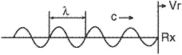# Doppler shift

Also found in: Dictionary, Thesaurus, Medical, Wikipedia.

## Doppler shift

See Doppler effect.

## Doppler shift

[′däp·lər ‚shift]
(physics)
The amount of the change in the observed frequency of a wave due to Doppler effect, usually expressed in hertz. Also known as Doppler frequency.

## Doppler shiftIn airborne Doppler radar, a transmitter which, by means of a directional antenna, radiates energy toward the ground. This results in a situation in which both the transmitter and the receiver are moving relative to the ground; consequently the original frequency is changed twice. The difference between transmitted and received frequencies is known as the Doppler shift and is very nearly proportional to the relative motion between the aircraft and the ground along the direction of the radar beam. A moving target will cause the frequency of the echo signal to increase if it is approaching the radar or decrease if it is receding from the radar. Since the Doppler frequency shift is proportional to radial velocity, a radar system that measures such a shift in frequency can also provide the radial velocity of a target. The Doppler frequency shift also is used to separate moving targets from stationary ones even when the undesired clutter power might be much greater than the power of echo from the targets. Also called the Doppler effect.

## Doppler effect

The change in electromagnetic frequency that occurs when the source of the radiation and its observer move toward or away from each other. The faster they come together, the higher the frequency. The faster they move away, the lower the frequency. Discovered by Austrian physicist Christian Doppler (1803-1853), this condition has a great effect on low-earth orbit (LEO) satellites as they weave towards and away from the earth. See Doppler radar.
References in periodicals archive ?
This is because the influence of Doppler Effect is getting weaker with the decreasing bandwidth which is the Delay Spread: Frequency Selective Fading and Doppler Shift. Based on the calculation, the higher the bandwidth, the stronger the Doppler Spread: Frequency Selective Fading and the Doppler Shift.
The parallel numbers of resampling units are controlled on frame-by-frame basis according to the measured Doppler shift mean and deviation.
The Doppler shift is determined by the user terminal access Elevation angel and the satellite tangential velocity, so Doppler shift in each LEO satellite channel multi-path can be approximated equal.
For flat fading channels without the effect of Doppler shift, the performance difference between OMP and MOMP is nearly invisible, which is shown in Figure 6.
where [MATHEMATICAL EXPRESSION NOT REPRODUCIBLE IN ASCII], denotes the maximum Doppler shift due to the transmitter (i = 1) or receiver (i = 2) which moves at velocity [v.sub.i] and carrier wavelength A.
Under a Rayleigh channel model, [P.sub.c] is calculated at different SNRs and different values of the Doppler shift of the channel.
A new method for estimating the Doppler shift and time delay of the overlapping echoes was presented by Qi et al.
Doppler shift caused by skin acceleration was recorded during each procedure.
In Sections 3-4, the analytical formulas for Doppler shift and bandwidth are derived when the effects of large-scale waves shadow and curvature are also taken into account.
The spacecraft made it half way across the solar system before engineers discovered a potentially fatal flaw in the communications system caused by dynamic signal distortions due to Doppler shift.' This problem limited the data bandwidth available to the probe, and its severity required engineers to alter the flight path of the spacecraft to work around the issue.
The Doppler shift estimations could be used for prerecorded vehicle pattern processing and for more complex learning algorithm solutions .
This frequency shift is called a Doppler shift. It is important to mention that the particles conveyed by a fluid flow to be measured must be big enough to scatter sufficient light for signal detection but small enough to follow the flow faithfully [Jafarzadeh, 2009].

Site: Follow: Share:
Open / Close